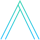# Introduction to Monte Carlo Methods

July 25, 2015

I’m going to keep this tutorial light on math, because the goal is just to give a general understanding.

Monte Carlo methods originated from the Manhattan Project, as a way to simulate the distance neutrons would travel through through various materials . Ideas using sampling had been around for a little while, but they took off in the making of the atomic bomb, and have since appeared in lots of other fields.

The idea is this:

Generate some random samples for some random variable of interest, then use these samples to compute values you’re interested in.

I know, super broad. The truth is because Monte Carlo has a ton of different applications, it’s hard to get a more precise definition. It’s used in product design, to simulate variability in parts manufacturing. It’s used in biology, to simulate average distance of a bird from it’s nest, which would allow a scientist to calculate energy expended. It can be used in AI for games, for example, the chinese game Go. And finally, in finance, to evaluate financial derivatives or option pricing . In short—it’s used everywhere.

The big advantage with Monte Carlo methods is that they inject randomness and real-world complexity into the model. They are also more robust to adjustments such as applying a distribution to the random variable you are considering. The justification for a Monte Carlo method lies in the law of large numbers. I’ll elaborate in the first example.

We can use something called the random darts method, a Monte Carlo simulation, to estimate pi. Here is my R code for this example.

The logic goes as follows—

If we inscribe a circle in a square, where one side length of the square equals the diameter of the circle we can easily calculate the ratio of circle area to square area.Now if we could estimate this value, we would be able to estimate pi.

We can do this by randomly sampling points in the square, and calculating the proportion of those inside the circle to the total points. So I just calculate red points over total points, and multiply by 4.We can see that as the number of points increases, the closer our value will get to pi. This is the law of large numbers at work.This is a simple example of a Monte Carlo method at work. We had some value we wanted to estimate, and we did so by generating a ton of random samples.

## Simulating Traffic

Here is a more useful and specific example. We can simulate traffic using the Nagel–Schreckenberg model. In this model, we have a road, which is made up by cells or spaces and contains a speed limit, and then a certain number of cars. We iterate through the cars and update their velocity based on the four following rules. Note – a car’s velocity = v.

1. Cars not at the maximum velocity will increase their velocity by one unit.
2. We then assess the distance d between a car and the car in front of it. If the car’s velocity is greater than or equal to the distance, we adjust it’s velocity to d-1.
3. Now we add some randomization. This is the step that makes it Monte Carlo-esque. With a probability p, we will reduce the cars velocity by 1.
4. Then, we move the car up v units.

View my code for getting the simulated data here, and for visualizing it in Processing here.I love how some of the “cars” are right on the bumper of the one in front of it, and others are just chilling out taking their time. Haha

## Challenges with Monte Carlo Methods

The first big challenge for Monte Carlo is how to come up with independent samples for whatever distribution your dealing with. This is a harder than you might think. In my code I just called R or Python’s built in random functions, but sampling can become much more sophisticated. That is a lot of what you will read about from more academic sources.

Here is a link on how R’s built in uniform sampling distribution works.

Another problem is getting the error to converge. Notice with the pi example how the error stopped converging quickly for the latter part of the graph. Most Monte Carlo applications just use really large samples due to low computing costs to compensate.

Monte Carlo methods are an awesome topic to explore, and I hope this post popularizes them even a little bit more (outside of finance and physics, that is).

## Sources

1. Wikipedia
2. Art Owen’s textbook on the subject. My favorite resource so far.
3. Kevin Murphy’s textbook.

# Join the mailing list

Get the latest posts in your inbox. No spam, ever.